# AP Board 9th Class Maths Solutions Chapter 1 Real Numbers Ex 1.1

AP State Syllabus AP Board 9th Class Maths Solutions Chapter 1 Real Numbers Ex 1.1 Textbook Questions and Answers.

## AP State Syllabus 9th Class Maths Solutions 1st Lesson Real Numbers Exercise 1.1

Question 1.
a) Write any three rational numbers.
Solution:
$$\frac{3}{4}, \frac{5}{9}, \frac{2}{7}$$

b) Explain rational number is in your own words.
Solution:
A number which can be expressed in algebraic form i.e., in $$\frac { p }{ q }$$ form is called a rational number.
E.g.: $$\frac { 3 }{ 5 }$$, $$\frac { -4 }{ 9 }$$ etc.Question 2.
Give one example each to the following statements.
i) A number which is rational but not an integer.
Solution:
7/11

ii) A whole number which is not a natural number.
Solution:
‘0’ (Zero)

iii) An integer which is not a whole number.
Solution:
-8

iv) A number which is natural number, whole number, integer and rational number.
Solution:
5

v) A number which is an integer but not a natural number.
Solution:
-4

Question 3.
Find five rational numbers between 1 and 2.
Solution: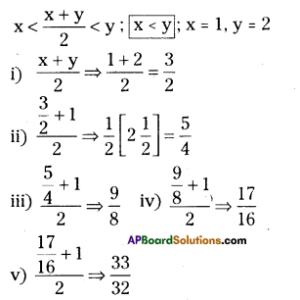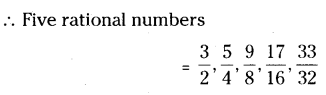Question 4.
Five rational numbers between $$\frac { 2 }{ 3 }$$ and $$\frac { 3 }{ 5 }$$
Solution: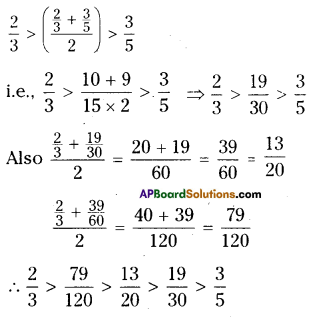Question 5.
Represent $$\frac { 8 }{ 5 }$$ and $$\frac { -8 }{ 5 }$$ on a number line.
Solution: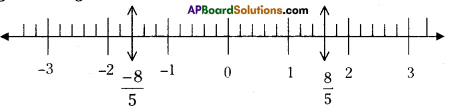Step – 1 : Draw a number line.
Step – 2 : Divide each unit into 5 equal parts.
Step – 3 : Take 8 – equal parts from ‘0’ on its right side and mark it as $$\frac { 8 }{ 5 }$$ (similarly) on left side $$\frac { -8 }{ 5 }$$ .

Question 6.
Express the following rational numbers as decimal numbers.
Solution:
I. i) $$\frac { 242 }{ 1000 }$$ .
ii) $$\frac { 354 }{ 500 }$$ .
iii) $$\frac { 2 }{ 5 }$$ .
iv) $$\frac{115}{4}$$
Solution:
i) $$\frac { 242 }{ 1000 }$$ = 0.242
ii) $$\frac{354}{500}$$
$$=\frac{354 \times 2}{500 \times 2}$$
$$=\frac{708}{1000}$$
$$=0.708$$
iii) $$\frac{2}{5}$$
$$=\frac{2 \times 2}{5 \times 2}$$
$$=\frac{4}{10}$$
$$=0.4$$
iv)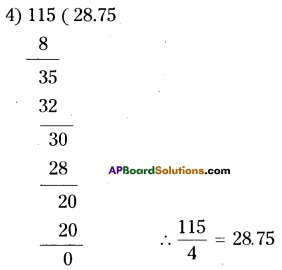II. i) $$\frac{2}{3}$$
Solution: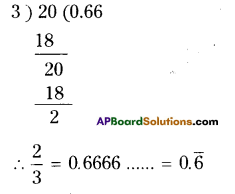ii) $$\frac{-25}{36}$$
Solution: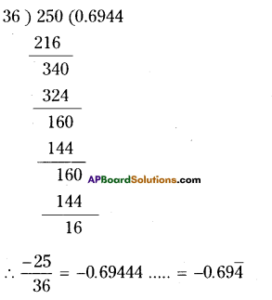iii) $$\frac{22}{7}$$
Solution: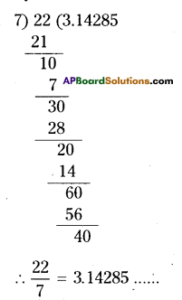iv) $$\frac{11}{9}$$
Solution: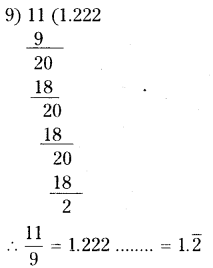Question 7.
Express each of the following decimals in $$\frac{p}{q}$$ form where q ≠ 0 and p, q are integers.
i) 0.36
Solution:
0.36 = $$\frac{36}{100}=\frac{9}{25}$$

ii) 15.4
Solution:
15.4 = $$\frac{154}{10}=\frac{77}{5}$$

iii) 10.25
Solution:
10.25 = $$\frac{1025}{100}=\frac{41}{4}$$

iv) 3.25
Solution:
3.25 = $$\frac{325}{100}=\frac{13}{4}$$

Question 8.
Express each of the following decimal number in the $$\frac { p }{ q }$$ form.
i) $$0 . \overline{5}$$
Solution:
Let x = $$0 . \overline{5}$$ = 0.5555
Multiplying both sides by 10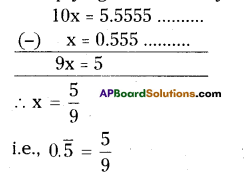ii) $$3 . \overline{8}$$
Solution:
Let x = $$3 . \overline{8}$$
(i.e) x = 3.888 ………..
Multiplying both sides by 10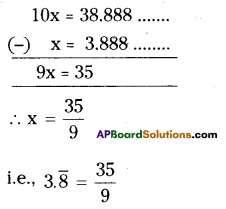iii) $$0 . \overline{36}$$
Solution:
Let x $$0 . \overline{36}$$
(i.e) x = 0.363636 ………..
Multiplying by 100 on both sides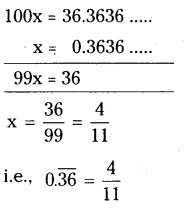iv) $$3.12 \overline{7}$$
Solution:
Let x = $$3.12 \overline{7}$$
x = 0.12777
Multiplying by 10 on both sides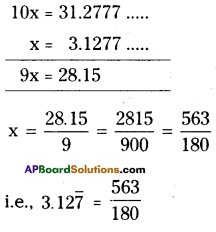Question 9.
Without actually dividing find which of the following are terminating
decimals.
i) $$\frac { 3 }{ 25 }$$
Solution:
Check the denominator, if it consists of 2’s or 5’s or combination of both then only it reduces to a terminating decimal.
25 = 5 x 5
Hence $$\frac { 3 }{ 25 }$$ is a terminating decimal.

ii) $$\frac { 11 }{ 18 }$$
Solution:
Denominator 18 = 2 × 3 × 3,
hence $$\frac { 11 }{ 18 }$$ is a non-terminating decimal 13

iii) $$\frac { 13 }{ 20 }$$
Denominator 20 = 2 × 2 × 5,
hence $$\frac { 13 }{ 20 }$$ is a terminating decimal.

iv) $$\frac { 41 }{ 42 }$$
Solution:
Denominator 42 = 2 × 3 × 7,
hence $$\frac { 41 }{ 42 }$$ is a non-terminating decimal.